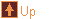Tensegrity Models 15 images tree :: Geometry > Tensegrity Models
In This Album:

 Image List Thumbnail Page
These tensegrity models have been made by connecting together edge units to form twisted polyhedra. The edge units have a notch at each end, and a loop of cord running along the length. They connect by slipping the cord of one edge into the notch of another. Each unit connects with four others: one at each notch and two along the cord. The basic idea is explained nicely by George Hart in his page on Soda Straw Tensegrity Structures.

I have built my units from two barbecue sticks bound by wire near each end, which gives the notches. The cord is either a loop of string, or a length of elastic knotted at each end and sliped through the notches.

The process of twisting transforms an equal edge polyhdron into an equal edge dual form. In the case of the regular and quasi-regular polyhedra that dual has the same shape as the "normal" dual (by polar reciprocation). Not all equal edge polyhedra have an equal edge twisted dual.

The regular and quasi-regular polyhedra are also linked with their twisting mid-point by a jitterbug-like transformation, as described in Polyhedral Twisters.

Twisted polyhedra are also popular as a base for wooden puzzles. A lot of these puzzles can be seen at Puzzle World.

 Image List

Octahedron  Med    Lrg
 Description : A twisted octahedron. The stuts come in four sets of three parallel struts which run along the middle of equilateral triangle channels. These correspond to the positions of a particular packing of rods with a triangular cross section. This helps make the twisted octahedron a popular base for wooden puzzles Example puzzlesOctahedron / Cube  Med    Lrg
 Description : The mid-point of twisting between an octahedron and cube. The cords form a cuboctahedron, having the six faces of the cube and 8 faces of the octahedron. The struts form four triangles inscribed in the equatorial hexagons of the cuboctahedron. Example puzzleCube  Med    Lrg
 Description : A twisted cube. Example puzzleCuboctahedron  Med    Lrg
 Description : A twisted cuboctahedron.Cuboctahedron / Rhombic Dodecahedron  Med    Lrg
 Description : The mid-point of twisting between a cuboctahedron and rhombic dodecahedron. The cords form a shape close to a small rhombicuboctahedron. It has the 14 faces of the cuboctahedron (6 squares and 8 triangles) and the 12 rhombi of the rhombic dodecahedron. The struts are arranged as 6 squares, arranged in parallel pairs offset by an 1/8 of a turn.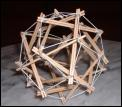Rhombic Dodecahedron  Med    Lrg
 Description : A twisted rhombic dodecahedron (viewed along a two-fold axis). Example puzzleRhombic Dodecahedron  Med    Lrg
 Description : A twisted rhombic dodecahedron (viewed along a four-fold axis). Example puzzlesIcosahedron  Med    Lrg
 Description : A twisted icosahedron. Like the twisted octahedron it has sets of parellel struts - in this case 5 sets of 6 struts. The triangular channels these run through are the shape of a golden gnomon, the triangle made from joining three consequetive points on a pentagon. This makes it another popular choice for wooden puzzles, as is described in this discussion of the Jupiter Example puzzlesIcosahedron / Dodecahedron  Med    Lrg
 Description : The mid-point of twisting between an icosahedron and dodecahedron. The cords form an icosidodecahedron, having the 12 faces of the dodecahedron and the 20 faces of the icosahedron. The struts form six pentagons inscribed in the equatorial decagons of the icosidodecahedron.Dodecahedron  Med    Lrg
 Description : A twisted dodecahedron.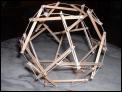Small Stellated Dodecahedron  Med    Lrg
 Description : A twisted small stellated dodecahedron (viewed along a five-fold axis). Example puzzles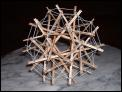Small Stellated Dodecahedron  Med    Lrg
 Description : A twisted small stellated dodecahedron (viewed close to a two-fold axis). Example puzzlesSmall Stellated Dodecahedron  Med    Lrg
 Description : A twisted small stellated dodecahedron (viewed close to a three-fold axis). One of the features of the twisted polyhedra is that each strut is paired with a parallel line on the other side of the centre through which lots of struts (possibly extended) pass. This feature is quite striking in this view. Three parallel struts are seen end-on, each paired with a line of crossing. Of the other struts, 24 pass through just one line, and three pass through two. Here is another example showing a crossing line in a twisted cube The property is taken good advantage of in this 30 Notched Sticks puzzle. The configuration of the pale sticks is a mirror image of that of the hexagonal sticks, with the two being related by a central inversion. Example puzzlesStella Octangula  Med    Lrg
 Description : A twisted stella octangula (viewed along a four-fold axis). The usual connection method was unstable in this polyhedron so the 8-way vertices have been connected slightly differently. If connected by the usual way the stella octangula would be the twisted dual of the truncated cube.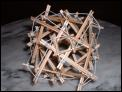Stella Octangula  Med    Lrg
 Description : A twisted stella octangula (viewed along a three-fold axis). The usual connection method was unstable in this polyhedron so the 8-way vertices have been connected slightly differently. If connected by the usual way the stella octangula would be the twisted dual of the truncated cube.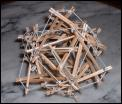Home   |   Programs   |   Examples   |   Album   |   Download   |   Development   |   Forum   |   AboutContact: adrian@antiprism.com      -      Generated on vie 21 oct 2016 08:52:40 CEST